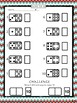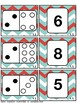# Pearson Realize Envision Topic 1 Centers, Activities, Resources for first gradeSubject
Resource Type
File Type

PDF

(17 MB|42 pages)
Product Rating
4.0
(6 Ratings)
Standards
• Product Description
• StandardsNEW

This is a Pearson Realize Envision packet with supplemental activities and math centers for Topic 1 first grade. 42 pages of resources!

This is something that I use in my 1st-grade classroom as center materials and independent work. These can be done after each lesson or repeated throughout the Topic (which is how I use it).

There are 8 differentiated worksheets and 5 center games.

These include:

-matching the number card to a model

-matching the number card to a model of the parts inside and outside

-Connect 4 addition number sentence game,

-mini flashcards

-word problem task cards (joining stories with result unknown, change unknown, and start unknown)

-recording sheets for all centers

+Plus a review or assessment page, depending on what you need

As well as ideas of how to use these resources in multiple ways :)

This product is segmented into activities for after suggested topic lessons. To make it easy for you, the activities are labeled by lesson on the bottom right corner of each page.

There is a guide before each set of lesson materials for further details and implementation ideas.

*******************************************************

--------------------Check out my other PEARSON products---------------------

Determine the unknown whole number in an addition or subtraction equation relating three whole numbers. For example, determine the unknown number that makes the equation true in each of the equations 8 + ? = 11, 5 = ▯ - 3, 6 + 6 = ▯.
Understand the meaning of the equal sign, and determine if equations involving addition and subtraction are true or false. For example, which of the following equations are true and which are false? 6 = 6, 7 = 8 - 1, 5 + 2 = 2 + 5, 4 + 1 = 5 + 2.
Apply properties of operations as strategies to add and subtract. If 8 + 3 = 11 is known, then 3 + 8 = 11 is also known. (Commutative property of addition.) To add 2 + 6 + 4, the second two numbers can be added to make a ten, so 2 + 6 + 4 = 2 + 10 = 12. (Associative property of addition.)
Use addition and subtraction within 20 to solve word problems involving situations of adding to, taking from, putting together, taking apart, and comparing, with unknowns in all positions, e.g., by using objects, drawings, and equations with a symbol for the unknown number to represent the problem.
Total Pages
42 pages
N/A
Teaching Duration
N/A
Report this Resource to TpT
Reported resources will be reviewed by our team. Report this resource to let us know if this resource violates TpT’s content guidelines.